Mr. Reece

•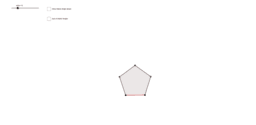Interior Angles in Regular Polygons

Activity

Mr. Reece

•Lines of Reflection

Activity

Mr. Reece

•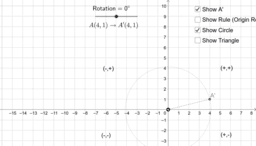Properties of Rotations

Activity

Mr. Reece

•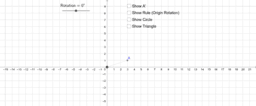Rotate Points

Activity

Mr. Reece

•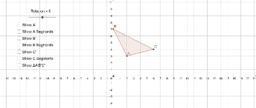Rotate Shapes

Activity

Mr. Reece

•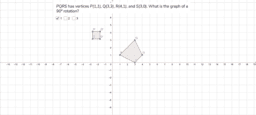Rotation Practice CO.1.2

Activity

Mr. Reece

•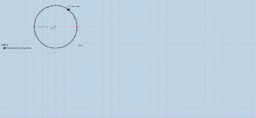Circle Equation: Center (h,k)

Activity

Mr. Reece

•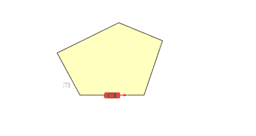Exterior Angles of Polygons

Activity

Mr. Reece

•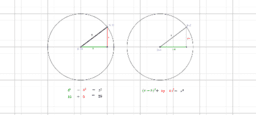Equation of Circle 2

Activity

Mr. Reece

•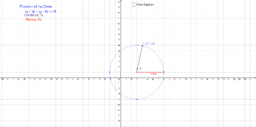equation of circle

Activity

Mr. Reece

•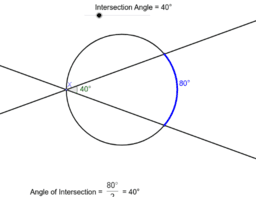Copy of Angle Measures from Intersection inside circle

Activity

Mr. Reece

•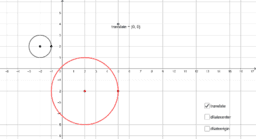Circle Transformations

Activity

Mr. Reece

•Exterior Convex Polygon Angle Theorem

Activity

Mr. Reece

•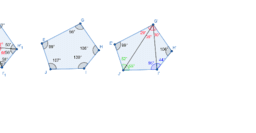Polygon Angle Sum Theorem

Activity

Mr. Reece

•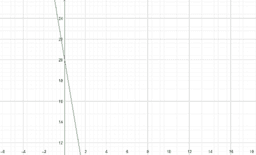Day 4 Linear Equations

Activity

Mr. Reece

•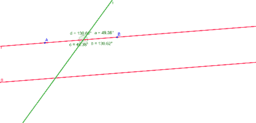Transversal

Activity

Mr. Reece

•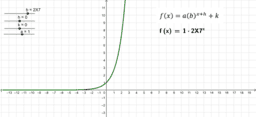Exponential Function Key Features

Activity

Mr. Reece

•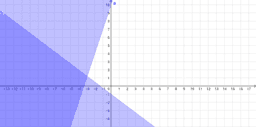Question 3

Activity

Mr. Reece

•Question 3

Activity

Mr. Reece

•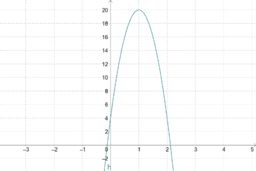4.1 Supplement Graphs

Activity

Mr. Reece

•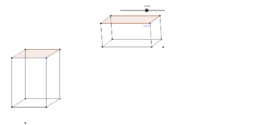Volume of a Prism and Cavalieri's Principle

Activity

Mr. Reece

•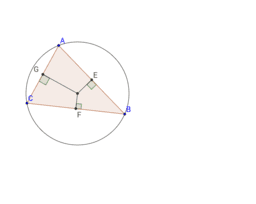Activity

Mr. Reece